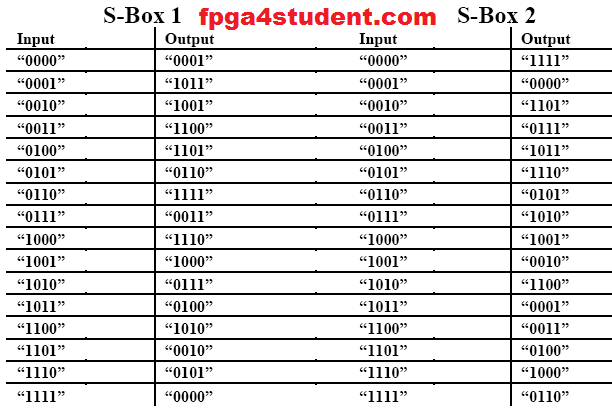# Non-linear Lookup Table Implementation in VHDL

## In this VHDL project, a nonlinear lookup table which is used in hashing functions of the upcoming co-processor is implemented in VHDL.

### The nonlinear operation which is used in hashing algorithm utilizes a parallel 4-bit nonlinear operation where the input nibble (4 bits) are mapped to another nonlinear 4-bit value.

#### The details of the lookup table implementation are as follows:### VHDL code for the nonlinear lookup table implementation:

```library IEEE;
use IEEE.STD_LOGIC_1164.ALL;
-- fpga4student.com: FPGA projects, Verilog projects, VHDL projects
-- VHDL project: VHDL implementation of Lookup Table
---------------------------------------------------
-- Non-linear Lookup Table Implementation in VHDL--
---------------------------------------------------
entity non_linear_lookup is
port (  LUTIN: in std_logic_vector(7 downto 0);
LUTOUT: out std_logic_vector(7 downto 0)
);
end non_linear_lookup;

architecture Behavioral of non_linear_lookup is
signal MSN_in,LSN_in,MSN_out,LSN_out: std_logic_vector(3 downto 0);
begin
MSN_in <= LUTIN(7 downto 4);
LSN_in <= LUTIN(3 downto 0);
SBOX_1: process(MSN_in) begin
case(MSN_in) is
when "0000" => MSN_out <= "0001";
when "0001" => MSN_out <= "1011";
when "0010" => MSN_out <= "1001";
when "0011" => MSN_out <= "1100";
when "0100" => MSN_out <= "1101";
when "0101" => MSN_out <= "0110";
when "0110" => MSN_out <= "1111";
when "0111" => MSN_out <= "0011";
when "1000" => MSN_out <= "1110";
when "1001" => MSN_out <= "1000";
when "1010" => MSN_out <= "0111";
when "1011" => MSN_out <= "0100";
when "1100" => MSN_out <= "1010";
when "1101" => MSN_out <= "0010";
when "1110" => MSN_out <= "0101";
when "1111" => MSN_out <= "0000";
when others => MSN_out <= "0000";
end case;
end process;
SBOX_2: process(LSN_in) begin
case(LSN_in) is
when "0000" => LSN_out <= "1111";
when "0001" => LSN_out <= "0000";
when "0010" => LSN_out <= "1101";
when "0011" => LSN_out <= "0111";
when "0100" => LSN_out <= "1011";
when "0101" => LSN_out <= "1110";
when "0110" => LSN_out <= "0101";
when "0111" => LSN_out <= "1010";
when "1000" => LSN_out <= "1001";
when "1001" => LSN_out <= "0010";
when "1010" => LSN_out <= "1100";
when "1011" => LSN_out <= "0001";
when "1100" => LSN_out <= "0011";
when "1101" => LSN_out <= "0100";
when "1110" => LSN_out <= "1000";
when "1111" => LSN_out <= "0110";
when others => LSN_out <= "0000";
end case;
end process;
LUTOUT <= MSN_out & LSN_out;
end Behavioral;```

### VHDL Testbench code for Nonlinear Lookup Table implementation:

```LIBRARY ieee;
USE ieee.std_logic_1164.ALL;
USE ieee.numeric_std.all;
-- fpga4student.com: FPGA projects, Verilog projects, VHDL projects
-- VHDL project: VHDL implementation of Lookup Table
-- Testbench VHDL code for Lookup Table Implementation
ENTITY tb_lookuptable IS
END tb_lookuptable;

ARCHITECTURE behavior OF tb_lookuptable IS

-- Component Declaration for Lookup Table Implementation in VHDL

COMPONENT non_linear_lookup
PORT(
LUTIN : IN  std_logic_vector(7 downto 0);
LUTOUT : OUT  std_logic_vector(7 downto 0)
);
END COMPONENT;

--Inputs
signal LUTIN : std_logic_vector(7 downto 0) := (others => '0');

--Outputs
signal LUTOUT : std_logic_vector(7 downto 0);
signal i: integer;

BEGIN

-- Instantiate the Lookup Table Implementation
uut: non_linear_lookup PORT MAP (
LUTIN => LUTIN,
LUTOUT => LUTOUT
);

stim_proc: process
begin
LUTIN <= x"00";
-- initialize 4-bit input data in VHDL testbench
for i in 0 to 15 loop
LUTIN <= std_logic_vector(to_unsigned(i, 8));
wait for 100 ns;
end loop;
wait;
end process;

END;```

#### Simulation waveform for the nonlinear lookup table in VHDL: